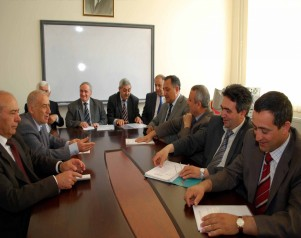﻿ BAKU STATE UNIVERSITY

## The chair of Differential and integral equationsThe chair of differential and integral equations was founded in 1958.

The first head of the chair was corresponding member of Azerbaijan National Academy of Sciences professor G.T.Ahmadov. Since 1975 till 2011 professor G.K.Namazov has been the head of the chair. From 2012 d.p.-m.s., professor N.Sh.Isgandarov is the head of this chair. At present the chair is functioning in the following content: d.p.-m.s., prof.N.Sh.Isgandarov  (dean of the faculty),  d.p.-m.s., prof.(consultant) M.A.Guliyev, c.p.-m.s., assoc.prof. M.I.Aliyev, c.p.-m.s., assoc.prof. A.S.Farajov, d.p.-m.s.,prof. Y.T.Mehraliyev (vice-dean of the faculty), c.p.-m.s., assoc.prof. A.I.Ismayilov, c.p.-m.s., assoc.prof. H.K.Musayev, c.p.-m.s., assoc.prof. Sh.H.Baghirov, c.p.-m.s., assoc.prof. M.B. Mursalova, c.p.-m.s.,Kh.T.Ismayilova, c.p.-m.s.,T.M.Gasimov, senior laboratory assistant Kh.A.Aliyeva.

The bachelors are taught the following subjects: Differential equations,  Equations of the mathematical physics, Asymptotic methods for differential equations, Partial differential equations of the first order, Integral equations. The undergraduate masters are taught the subjects: Contemporary problems of science (on the corresponding branch), The course recommended to universities and colleges, The Cauchy problem and boundary-value problems for differential equations in  partial derivatives, Theorems of embedment and their applications, Inverse problems of mathematical physics, Theory of thermal conductivity and its applications ,Additional chapters of differential equations, Spectral theory of differential operators, Boundary-value problem for linear equations of higher order.

The chair collaborators are authors of over 1000 scientific papers, tens of monographs, textbooks, methodic books. About 60 candidate and 8 doctorial thesis where defended under the supervision of the chair collaborators.

The direction of scientific investigations conducted at the chair are study of the questions of existence and uniqueness of solution of some class of initial, boundary and inverse problems for differential equations in partial derivatives and problems of optimal control.

Employments:

Mehraliyev Ya.T. - chief of the chairprofessor, doctor of physical and mathematical sciences,

Isgenderov N.Sh.- dean, professor, doctor of physical and mathematical sciences

Guliyev M.A.- (consultant),professor, doctor of physical and mathematical sciences

Aliyev M.I. - associate professor, candidate of physical and mathematical sciences

Farajov A.S. - associate professor, candidate of physical and mathematical sciences

Ismailov A.I. - associate professor, candidate of physical and mathematical sciences

Musayev H.K. - associate professor, candidate of physical and mathematical sciences

Bagirov Sh.H. - associate professor, candidate of physical and mathematical sciences

Mursalova M.B. - associate professor, candidate of physical and mathematical sciences

Huseinova Kh.T.- lecturer, candidate of physical and mathematical sciences

Gasimov T.M.-lecturer ,doctor philosophy in mathematics

Taught the main discipline:

Bachelors:

Specialty: mathematics

Ordinary differential equations 1-2

Equations of the mathematical physics

Partial differential equations of the first order

Asymptotic methods for differential equations

Specialty: teacher of mathematics

Ordinary differential equations

Equations of the mathematical physics

Integral equations

Specialty: mechanics

Ordinary differential equations

Equations of the mathematical physics

Magistracy:

Contemporary problems of science (on the corresponding branch)

The Cauchy problem and boundary-value problems for differential equations in partial derivatives

Theorems on embedment and their application

Inverse problems of mathematical physics

Theory of thermal conductivity and its applications